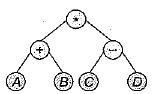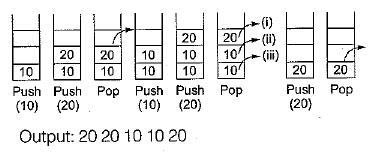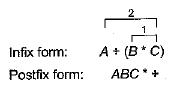Courses

# Test: Arrays, Stack, Queues & Linked List- 2

## 15 Questions MCQ Test Question Bank for GATE Computer Science Engineering | Test: Arrays, Stack, Queues & Linked List- 2

Description
This mock test of Test: Arrays, Stack, Queues & Linked List- 2 for Computer Science Engineering (CSE) helps you for every Computer Science Engineering (CSE) entrance exam. This contains 15 Multiple Choice Questions for Computer Science Engineering (CSE) Test: Arrays, Stack, Queues & Linked List- 2 (mcq) to study with solutions a complete question bank. The solved questions answers in this Test: Arrays, Stack, Queues & Linked List- 2 quiz give you a good mix of easy questions and tough questions. Computer Science Engineering (CSE) students definitely take this Test: Arrays, Stack, Queues & Linked List- 2 exercise for a better result in the exam. You can find other Test: Arrays, Stack, Queues & Linked List- 2 extra questions, long questions & short questions for Computer Science Engineering (CSE) on EduRev as well by searching above.
QUESTION: 1

### The information about an array that is used in a program wiil be stored in

Solution:

A dope vector is a data structure used to hold information about a data objects eg. an array, especially layout of array’s memory. It contain information such as rank of an array, type of elements of array etc.

QUESTION: 2

### Which of the following expressions accesses the (i, j)th entry of a (m x n) matrix stored in column major form?

Solution:

(i, j) entries in column major order of size (m x n).
Assume starting address is 1 so, m x (j - 1) + i

QUESTION: 3

### Sparse matrices have

Solution:

Sparse matrices are those matrices in which most of the elements are zero.
In contrast, if most of the elements are non-zero then the matrix is considered DENSE.

QUESTION: 4

The postfix equivalent of the prefix * + ab - cd is

Solution:

The tree whose preorder traversal yields * + AB - CD, is given in figure. Write the postorder traversal of the tree. That is the postfix form.Postorder traversal = AB + CD-*

QUESTION: 5

Stacks cannot be used to

Solution:

Stacks are used to evaluate postfix expressions, to store data in case of recursion and to convert infix forms to postfix forms.
However, resource allocation can’t be done using stack. Scheduling algorithms are used for allocation of CPU time

QUESTION: 6

The postfix expression for the infix expression: A + B* (C + D) / F + D * E is:

Solution:

Infix: A + B *(C + D)/ F + D *E
Postfix: ABCD + *F/ + DE* +

QUESTION: 7

In a circularly linked list organization, insertion of a record involves the modification of

Solution:

For insertion/deletion of a record in circularly linked list involves 2 pointer.
But for reverse the linked list, it involves 3 pointer.

QUESTION: 8

Stack is useful for implementing

Solution:

Stack is used for implementing recursion and depth first search.
For breadth first search, queue is used.

QUESTION: 9

Which of the following is useful in implementing quick sort?

Solution:

Immediately after visiting a node, append it to the queue. After visiting all its children, the node currently in the head of the queue is deleted. This process is recursively carried out on the current head of the queue, till the queue becomes empty.

QUESTION: 10

The process of accessing data stored in tape is similar to manipulating data on a

Solution:

The process of accessing data stored on tape is similar to manipulating data on a set.

QUESTION: 11

Linked lists are not suitable for implementing

Solution:

In case of using link list, one cannot randomly access the data or only serial data access is there. But, in case of binary search one needs to jump randomly either to first half or other half, which is not possible with linked list.

QUESTION: 12

The following sequence of operations is performed on a stack,
PUSH(10), PUSH (20), POP, PUSH (10), PUSH (20), POP, POP, POP, PUSH(20), POP
The sequence of values popped out is

Solution:QUESTION: 13

Which of the following operations is performed more efficiently by double linked list than by linear linked list?

Solution:

In linear linked list we can search only in one way but using double linked list we can search an unsorted list for a given item in both direction.

QUESTION: 14

The smallest element of an array’s index is called its

Solution:

Smallest element of an array’s index is called its LOWER BOUND and largest array index is called its UPPER bound.

QUESTION: 15

The post fix form A + (B* C) is

Solution: